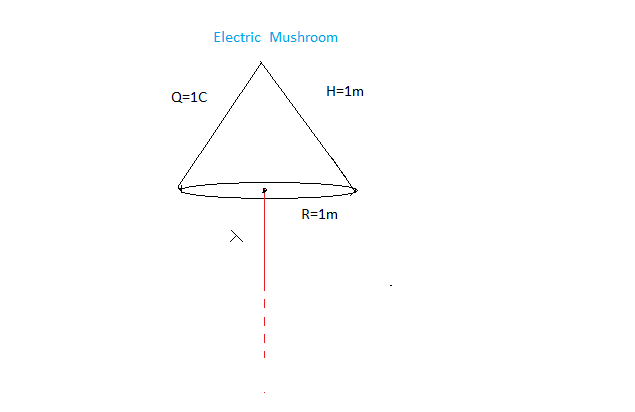# Electric Mushroom !Let a solid uniformly charged cone having total charge $Q$, radius $R$ and height $H$ is kept in contact with uniformly charged wire having charge per unit length $\lambda$ as shown. Then calculate the electric interaction force between them and report your answer to nearest integer.

Details and Assumptions

\begin{aligned} Q & = & 1 C \\ R & = & 1m \\ H & = & 1m \\ \lambda & = & 10^{-9} Cm^{-1} \\ \frac {1}{4\pi \epsilon} & = & 9 \times 10^9 Nm C^{-2} \\ \end{aligned}

×